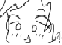Discussion about math, puzzles, games and fun.   Useful symbols: ÷ × ½ √ ∞ ≠ ≤ ≥ ≈ ⇒ ± ∈ Δ θ ∴ ∑ ∫  π  -¹ ² ³ °

You are not logged in.

## #1 2020-09-11 12:04:37

Mathegocart
MemberRegistered: 2012-04-29
Posts: 2,073

### Summation - pretty easy..

Hello MIF friends,

I appear to be having some difficulty with some (admittedly pretty simple sums)..So..

1. Sum of 1 from i=0 to N: seems easy enough, 1 + N = N+1.

2. Sum of 1 from t=1 to T. T. Now sum it up from k=1 to k=K, and you have.. TK.

3. Now this one is where I'm seemingly having some difficulty:

the sum of 0.5^k from t=1 to t=T is 1 - 2^(-T) (as per the geometric summation formula.) now sum that up from k=1 to K, and we have.. K(sum of 1 from 1 to K) + (2^ (-K) - 1)(as per a reapplication of the geo. sum formula.) I think this is right.. could you guys confirm?

4. Now this is in a similar mold as the last problem - the sum of 0.5^k from t=1 to t=T is 1 - 2^(-T).. but I think that when you sum that from k=1 to inf, it diverges. Is that true?

Thank you.

Last edited by Mathegocart (2020-09-11 12:05:28)

The integral of hope is reality.
May bobbym have a wonderful time in the pearly gates of heaven.
He will be sorely missed.

Offline

## #2 2020-09-11 12:20:12

Mathegocart
MemberRegistered: 2012-04-29
Posts: 2,073